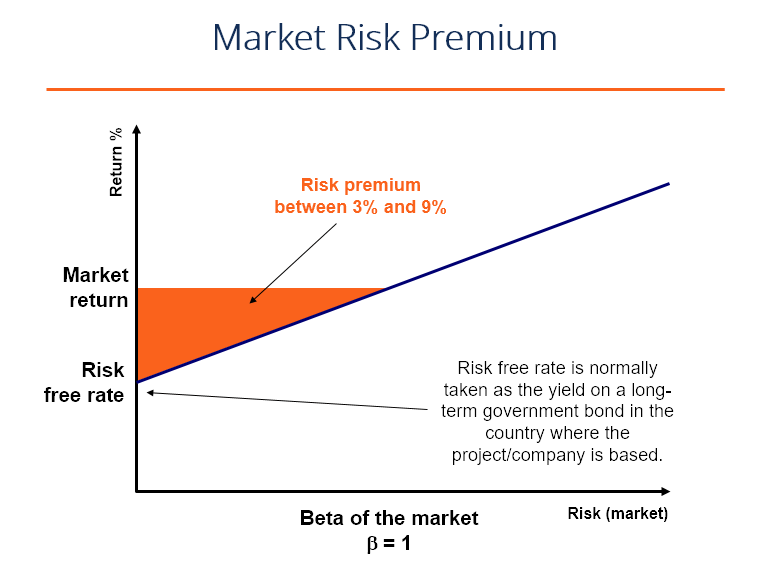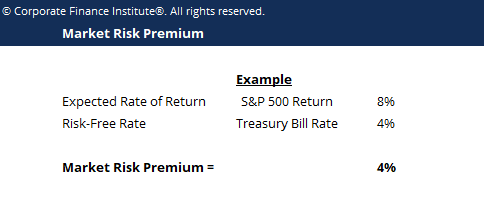A level of return a market generates that exceeds the risk free rate

## What is the Market Risk Premium?

The market risk premium is part of the Capital Asset Pricing Model (CAPM) which analysts and investors use to calculate the acceptable rate of return.  At the center of the CAPM is the concept of risk (volatility of returns) and reward (rate of returns).  Investors always prefer to have the highest possible rate of return combined with the lowest possible volatility of returns.### Concepts Used to Determine Market Risk Premium

There are three concepts related to the market risk premium:

1. Required market risk premium – the minimum amount investors should accept. If an investment’s rate of return is lower than that of the required rate of return, then the investor will not invest. It is also called hurdle rate of return.
2. Historical market risk premium – a measurement of the return’s past investment performances taken from an investment instrument that is used to determine the premium. The historical premium will produce the same result for all investors as the value’s calculation is based on past performances.
3. Expected market risk premium – based on the investor’s return expectation.

The required and expected market risk premiums would differ from one investor to another. During the calculation, the investor needs to take the cost of equity it takes to acquire the investment into consideration.

With a historical market risk premium, the return will differ depending on what instrument the analyst uses. Most analysts use S&P 500 as a benchmark for calculating past performance.

Usually, a government bond yield is the instrument used to calculate risk-free assets as it has little to no risk.

### Market Risk Premium Formula & Calculation

The formula for Market Risk Premium is:

Market Risk Premium = Expected Rate of Return – Risk-Free Rate

Example:

S&P 500 generated a return of 8% the previous year, and the current rate of the Treasury bill is 4%. The premium is 8% – 4% = 4%.### Use of Market Risk Premium

As stated above, the market risk premium is part of the Capital Asset Pricing Model. In the CAPM, the return of an asset is the risk-free rate plus the premium multiplied by the beta of the asset. The beta is the measure of how risky an asset is compared to the market, and as such, the premium is adjusted for the risk of the asset. An asset with zero

An asset with zero risk, and therefore zero beta, for example, would have the market risk premium canceled out. On the other hand, a highly risky asset, with a beta would 0.8, would take on almost the full premium.  At 1.5 beta, the asset is 150% more volatile than the market.

### Volatility

It’s important to reiterate that the relationship between risk and reward is the main premise behind market risk premiums.  If a security returns 10% every time period without fail, it has zero volatility of returns.  If a different security returns 20% in period one, 30% in period two, and 15% in period three, it has a higher volatility of returns and is therefore considered “risker”, even though it has a higher average return profile.

We hope this has been a helpful guide to understanding the relationship between risk and reward in corporate finance. CFI is the official global provider of the Financial Modeling and Valuation Analyst (FMVA) certification program.  To keep learning more about corporate finance and financial modeling, we suggest reading the articles below to expand your knowledge base.

• Weighted Average Cost of Capital WACC
• Sharpe Ratio Calculator
• Valuation Methods
• Valuation Infographic

### Financial Analyst Training

Get world-class financial training with CFI’s online certified financial analyst training program!

Gain the confidence you need to move up the ladder in a high powered corporate finance career path.

Learn financial modeling and valuation in Excel the easy way, with step-by-step training.